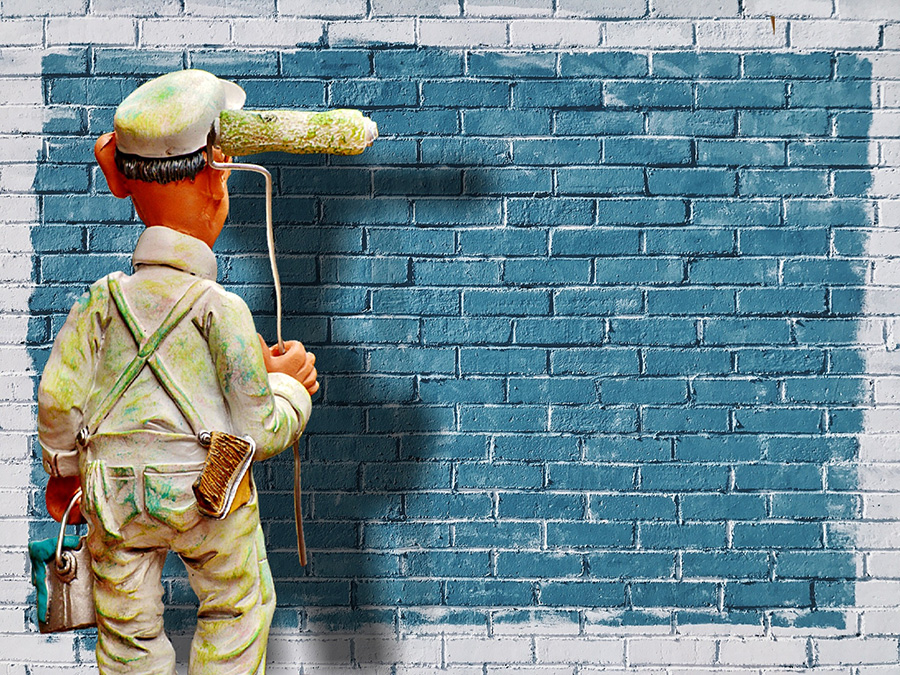# Paint Calculator

Estimate how much paint in gallons or litres you would need to paint a room or external surface. This paint calculator supports doors and windows, number of coats and custom paint coverage efficiency. Internal painting calculation excludes the ceiling.

Paint characteristics

m2/litre
\$
Share calculator:

Embed this tool:
get code

## Calculating how much paint you need

In order to calculate how many gallons or litres of paint you need to paint a room, house, or an exterior surface, you need to either know the measurements by plan, or have a long measurement tape ready (10 or 20 meter / 33 or 65 foot long is advised) before you can use this paint calculator. If you are painting an interior surface, you need to know the room's width, length and height. Rooms with non-rectangular layout can be approximated to rectangles. If you need to paint a ceiling or external wall, switch to "External" in the calculator and just enter the total width and height of the wall (or width and length instead, for a ceiling).

Usually the room or exterior will have openings for windows and doors which need to be excluded from the total surface area. Accurate measurement of the windows is important, especially if there are many of them. Our tool only supports one set of window dimensions, so if you have a room with multiple windows of highly different dimensions, you'd need to use the average. In this how much paint do I need calculator door dimensions are assumed to be close to construction standards (200mm by 90mm).

The other important thing to consider is the paint coverage efficiency (a.k.a. spreading rate): how many square meters or square feet you can cover with a litre or gallon of the paint. Manufacturers usually provide this information on the product label. Different paints and primers have different coverage capacity so make sure you use the right number as input. You'd want to allow for a lower efficiency in case the surface is not smooth. More coarse surfaces require more paint to cover.

Finally, if you enter the paint price per gallon or per litre the output will include the total price of the paint required. The tool rounds up to the closest gallon or litre, but you might want to order more paint just to be on the safe side.## Paint calculation example

One needs to paint two rooms top to bottom, excluding the ceiling and needs to know how many gallons of paint to purchase. Both rooms have two windows each 3 feet wide by 4 feet high. Each room also has a door with standard dimensions (19.4 square feet or 1.8 square meters). The dimensions of the first room are 12 feet by 15 feet, and the second is 12 feet by 18 feet. Both are 8 feet high. What amount of paint is needed to cover all walls with two coats of paint?

First, calculate the total internal surface area of the rooms: (8 x 12) x 2 + (8 x 15) x 2 = 192 + 240 = 432 square feet for the first one; (8 x 12) x 2 + (8 x 18) x 2 = 192 + 288 = 480 square feet for the second room. In total: 912 square feet.

Second, calculate the total surface area of the windows: 4 x (3 x 4) = 48 sq ft. and then add the two doors: 48 + 2 x 19.4 = 86.8 sq ft.

Third, subtract the surface area of the windows and doors from the total initially calculated to get how the paint needs to cover: 912 - 86.8 = 825.2 square feet.

Finally, if the paint is known to cover 108 ft2 per gallon, and given that two coats are needed, divide the square footage by the paint coverage, then multiply by the number of coats to get: (825.2 / 108) x 2 = 7.64 x 2 = 15.2 gallons needed to paint these two rooms. Since usually you can purchase paint in whole gallons only, and also since one should always buy a bit more to be on the safe side, 16 gallons of paint need to be bought for this interior painting project.

#### Cite this calculator & page

If you'd like to cite this online calculator resource and information as provided on the page, you can use the following citation:
Georgiev G.Z., "Paint Calculator", [online] Available at: https://www.gigacalculator.com/calculators/paint-calculator.php URL [Accessed Date: 01 Apr, 2023].